## Friday, 14 January 2011

### "You Ain't Seen Nothing Yet", But You Might Soon

Apologies to Bachman Turner Overdrive...
In my recent blog about the solution to a variation of the birthday problem I promised to expand on the idea that if you haven't witnessed an occurrence of an event in a large (large enough) number of multiple trials, then we can say with confidence (95% statistically) that the probability of success will be no greater than 3/n. This is often called the "statistical rule of three."

If a binomial event has a probability of p, then the probability that after n trials there are no successes will be (1-p)n, you have to have a non-success every trial. And if you want that probability to be less than .05, then we have an exponential equation to solve,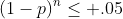We take the natural log of both sides to make the problem simpler to solve,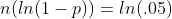Now if you have been wondering about where the three gets into it, this is it... the ln of .05 is very nearly -3. This gives us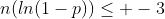. Now we have to do a little calculus approximation. For very small values of p, ln(1-p) is very close to -p. Which means our equation is almost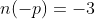..and dividing we can arrive at p=3/n.
So if we collect give 30 people an injection and none of them can experience a side effect, the rule of three suggests that we can be pretty (95%) sure that the true probability of the side effect is no greater than 1/10.

It seems to me that this approach is looking at the problem a little wrong. Let me try to explain. Let's take a very simple problem. Suppose we have a machine that spits out two ping-pong balls every time you press a button. The machine has five settings inside that control the probability that the ping-pong balls are white (otherwise they are some other color). The probabilities are equally spaced, 0, 1/4, 1/2, 3/4, and 1.
We want to know how how frequently we might get no white balls when we push the button. We make a table of the possible settings, and the probability that both balls are non-white.Since the machine settings are random each of them has a probability of 1/5. To find the total probability of getting no whites, we multiply 1/5 times each probability and add them up. (1/5)(1)+(1/5)(9/16)+(1/5)(1/4)+(1/5)(1/16)+(1/5)(0)= 3/8. We would expect that, on average, 3/8 of the times we run the machine, we get two non-white balls.

But there is a different question that can be asked: "if someone got two non-white balls, what is the probability that the machine was set at some particular value (say p=1/2). This is the "conditional probability" that seems to make students crazy. But in this situation it look pretty clear. Of 80 people who ran the machine, 30 of them got two non-white balls. If we look just at this 30 people, we would see that only 1 of them had occurred when the machine was set to p=3/4, 4 when it was set to p=1/2, and the other 25 occurred when the machine was set to p=1/4 or p=0.

Notice that we can say two very similar sounding things that are quite different.
1) The probability you get two non-white balls IF the machine probability of white is set to p=1/2 or greater is less than 1/4. (I think this is the approach the rule of three uses)
2) The probability the machine is set to p(white)=1/2 or higher IF you get two white balls is 5/30 or 1/6. (I think this is the way the confidence interval should be calculated.)

Now if we extend the number of options of the machine probability of white to any real number in the interval 0 to 1, then we can answer the same questions using integration to find the area under the curve (1-p)2 to give us the probability of getting two non-white balls. It turns out to be 1/3.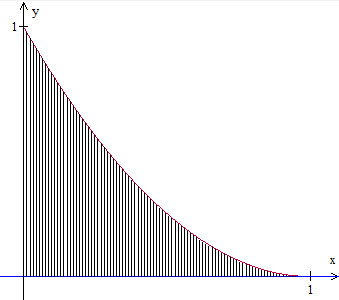Now we can answer the same two questions as above
1) The probability you get two non-white balls IF the machine probability of white is set to p=1/2 or greater is less than 1/4. (This is exactly the same as before, we are just asking what is the function value when the input is 1/2)
2) The probability the machine is set to p(white)=1/2 or higher IF you get two white balls is the ratio of the shaded area to the right of x=1/2 to the total shaded area. It turns out that in this case (two failures) we get about .043/(1/3) or about 12%.

So what if we got three or four or more failures. The graph starts to look more and more skewed as the probability of failure clumps to the left of the curve. Here is the graph of (1-p)5 in red, and(1-p)10 in blue .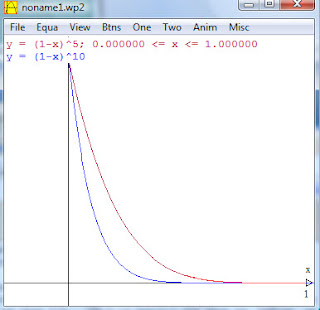Our rule of three says that if we got ten consecutive failures, we can be pretty sure that the prob of success is probability greater than 3/10. I turns out that for p=3/10, the probability of getting ten failures in a row is about 2.8%, and even lower for any probability greater than 3/10. This suggests that even for relatively small values of n, the limit of 3/n is a conservative approach to bounding the probability of an occurrence. But is it the best estimate of the probability given that we have ten failures. Given that we have had ten failures, the probability that the success probability was 3/10 or higher is the ratio of the area under (1-p)10 between 3/10 and 1 (about .00179) over the area between 0 and 1 (that's 1/11 or .0909090..). The overall ratio is a little less than 2%.

The conclusion for me? The rule of three is a very over-cautious estimate, but well worth the effort when you consider the ease of computation.Anonymous said...

There's a Bayesian approach to the problem you may find more satisfying.
You have an unknown parameter, the probability of success (call it s).

You want to know what the probability distribution for s is after observing the data (which happens to be n failures). This posterior distribution depends on 2 things: the likelihood function (probability of observed data given a particular value of s) and the prior distribution of s (what you would believe with no data).

Since the likelihood function is the binomial (probability of observing k successes is (n choose k) s^k (1-s)^(n-k) ), the popular choice for modeling the prior and posterior is the beta distribution.
In fact, the really popular model for the prior is beta(1,1) which makes all values of s equally likely.

The posterior probability distribution after n trials in which there are k successes is beta(k+1, n-k+1), and you can compute the mean a posteriori estimate of s as (k+1)/(n+2).
So if there are no successes in n trials, the maximum likelihood estimate for s is 0, and the MAP estimate is 1/(n+2).

Disclaimer: I'm a bioinformatician, not a Bayesian statistician, and so my explanation of the mechanism for using pseudocounts may not be as rigorous as a statistician would like.

Pat's Blog said...

Gas..
So would a Bayesian make anything like a confidence interval statement? If the MAP is a "mode" does that mean they would argue the rule of three was too liberal?

Thanks for the contribution.